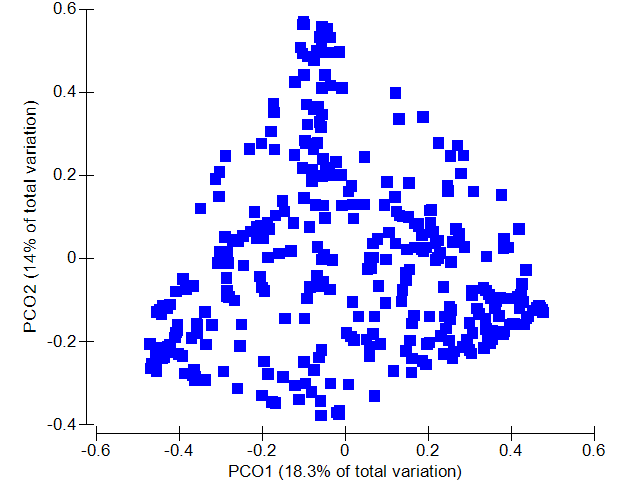# Eigenvalues generated by qiime2 are different from ape packages and PRIMER-e software

Hi all,

As we know, PCoA is one type of eigenanalysis. Each PCo is associated with an eigenvalue. The sum of the eigenvalues will equal the sum of the variance of all variables in the data set. The relative eigenvalues thus tell how much variation that a PCo is able to ‘explain’.Axes are ranked by their eigenvalues. Thus, the first axis has the highest eigenvalue and thus explains the most variance, the second axis has the second highest eigenvalue, etc.

However, I recently found that Eigenvalues that generated by Qiime2, R package "ape" and PRIMER-e software are different.

For consistency, I used the same dataset (bray-curtis):

1. R: import relative abundance table, and generate bray-curtis dissimilarity indices using vegdist() in vegan package and acquire eigenvalues/relative eigenvalues using pcoa() function from age package

2. Qiime 2: obtained bray-curtis distance matrix and then convert it to qzv and view it from qiime 2 view

3. PRIMER: import bray-curtis distance matrix, then PCoA it

My questions:

1. Could anyone help explain what eigenvalues does qiime2 use? Eigenvalues or Relative eigenvalues or Relative eigenvalues after Lingoes or Cailliez correction?
2. Could anyone let me know which result I should trust? Qiime2 or ape or PRIMER-e considering the results are not inconsistent.
3. From the options I found in pcoA() function in ape package, there are many values I can extract from the result list: Eigenvalues, Relative eigenvalues, Corrected eigenvalues (Lingoes correction) and Relative eigenvalues after Lingoes or Cailliez correction. If ape package is right one to choose, which value "PCo1 (? %)" should be used in the figure? My understanding is relative eigenvalues, but I am not sure whether Relative eigenvalues after Lingoes or Cailliez correction is more accurate to explain the variance of microbiome.

Attached are three different outcomes:

1. Eigenvalues/Relative eigenvalues from ape package
1. "Eigenvalues" from Qiime 2 (not sure it is Eigenvalues or Relative Eigenvalues)
1. "Eigenvalues" from PRIMER (not sure it is Eigenvalues or Relative Eigenvalues)
• Axis 1: ~18.3% / Axis 2: ~ 14%Last but not the least, thank you in advance for helping me solve my questions

Best Regard,
Godric

1 Like

From the docs, "By default, uses the default eigendecomposition method, SciPy's `eigh`, which computes all eigenvectors and eigenvalues in an exact manner."

Here's the SciPy docs on `eigh()`, which does not seem to mention relative results or correction of any sort, which is pretty different than the many options provided by `ape`.

I trust a result if I understand the method that made it, which is why this question is so important!

I also thought Emperor plots showed relative eigenvalues, but I can't find the code where these are calculated from the uncorrected eigenvalues. Are we looking in the right place?Lets see if other folks know more!

3 Likes

The SciPy documentation you cite does not mention distance (or dissimilarity) matrix, but seems to refer to crossproducts (which are complements of distances). To use that, you should transform your dissimilarities to cross-product like entities. Gower explained how to do this, and this is done in PCoA functions. What it really does is more than I care to look at, but straight eigh() for dissimilarities seems not be what you should do.

Then about relative or absolute ev's: if you have percentages (%), it must be relative. Relative to what depends on the software you use. Common choices are relative to the trace and relative to the sum of positive eigenvalues. The first case will give total percentages >100% for positive eigenvalues (negative eigenvalues will fix this to 100%), and the latter case will give 100% for positive eigenvalues, but will ignore negative eigenvalues.

I personally have no idea what to do with eigenvalues so I don't give any advice on them. I just think that eigenvalues are pretty useless.

4 Likes

I agree with @colinbrislawn and @Jari_Oksanen - I don't have much else to add, except a brief question about your process. Are you making sure to use the exact same feature table as the starting point in each of your test cases? You haven't told us what q2-diversity commands you're running - the pipelines for `core-metrics` and `core-metrics-phylogenetic` both include a rarefaction step, which adds an element of randomness to the resulting dataset.

2 Likes